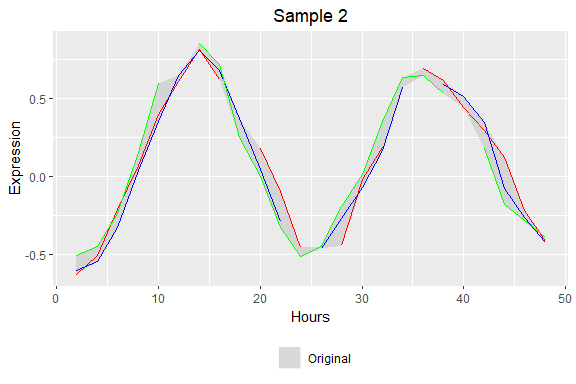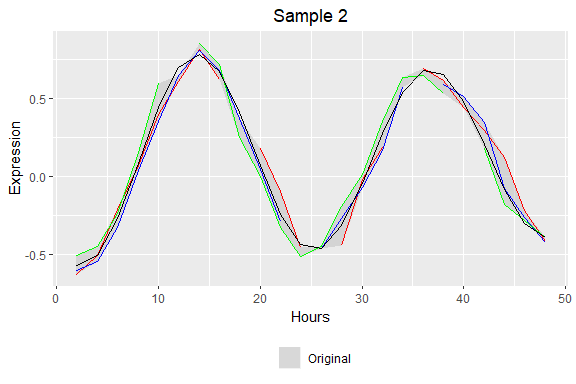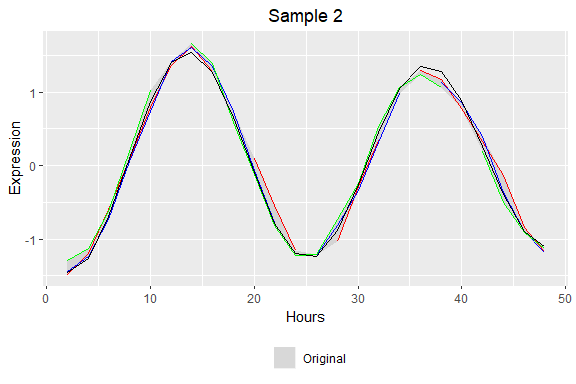# Introduction to Using ECHO

#### 2018-12-05

The echo.find package provides a function (echo_find()) designed to find rhythms from expression data using extended harmonic oscillators. To read more about our inital work on this project and cite us, see Circadian Rhythms in Neurospora Exhibit Biologically Relevant Driven and Damped Harmonic Oscillations by H. De los Santos et al. (2017) Further, for users who prefer an interface more than coding, as well as built-in visualizations, our GitHub repository can be found here. There, you can find a shiny application for finding rhythms and automatically visualizing results, with features such as Venn diagrams, heat maps, gene expression plots (with or without replicates visualized), and parameter density graphs. A FAQ for possible user errors can also be found there.

In this vignette, we’ll walk through an example of how to use echo.find, and how to choose from the several different built-in methods of preprocessing.

## Loading and Examining Data

We’ll start by loading our library, which contains the echo_find() function. It also has an example dataframe, expressions, which we’ll be using throughout this vignette. Here we’ll look at the first few rows and columns of our dataset.

library(echo.find)
head(expressions[,1:5])
##   Gene.Name      CT2.1      CT2.2      CT2.3      CT4.1
## 1  Sample 1  1.6331179  1.4976053  1.5138102  1.3095535
## 2  Sample 2 -0.6303192 -0.6027464 -0.5105009 -0.5062033
## 3  Sample 3         NA -0.7802214 -0.7767950  0.2847617
## 4  Sample 4  0.4659923  0.4940659         NA  0.1018655
## 5  Sample 5  0.7026372  0.6405812  1.0235155  1.7199453
## 6  Sample 6  0.9261508  0.8858768  0.8035570         NA

Note the data format: its first column first column has gene labels/names, and all other columns have numerical expression data. This expression data is ordered by time point then by replicate, and has evenly spaced time points. Any missing data has cells left blank. In order to use the echo_find() function, data must be in this format. Now, let’s look at one the data expressions, Sample 2. Here we plot each of the replicates in a different color, then plot the difference between them in gray.

library(ggplot2)

tp <- seq(2,48,by=2) # our time points
num_reps <- 3 # number of replicates

samp <- 2 # sample we want to look at
ex.df <- expressions[samp,-1] # expression data for the first sample

# our visualization data frame
ribbon.df <- data.frame(matrix(ncol = 3+num_reps, nrow = length(tp)))
# assigning column names
colnames(ribbon.df) <- c("Times","Min","Max",
paste(rep("Rep",num_reps),c(1:num_reps), sep="."))
ribbon.df$Times <- tp # getting min values of replicates ribbon.df$Min <- sapply(seq(1,ncol(ex.df), by = num_reps),
function(x) min(unlist(ex.df[,c(x:(num_reps-1+x))]), na.rm = TRUE))
# getting max values of replicates
ribbon.df$Max <- sapply(seq(1,ncol(ex.df), by = num_reps), function(x) max(unlist(ex.df[,c(x:(num_reps-1+x))]), na.rm = TRUE)) # assign each of the replicates to the visualization data frame for (i in 1:num_reps){ ribbon.df[,3+i] <- t(ex.df[,seq(i,ncol(ex.df),by=num_reps)]) } # color names color_bar <- c("Rep.1"="red","Rep.2"="blue","Rep.3"="green") # visualize, with shading for each row p <- ggplot(data = ribbon.df,aes(x=Times))+ # declare the dataframe and main variables geom_ribbon(aes(x=Times, ymax=Max, ymin=Min, colour="Original"), fill = "gray", alpha = 0.5)+ # create shading ggtitle(expressions[samp,1])+ # gene name is title scale_color_manual("",values=color_bar)+ scale_fill_manual("",values=color_bar)+ theme(plot.title = element_text(hjust = .5), legend.position = "bottom",legend.direction = "horizontal")+ labs(x="Hours", y="Expression") #Label for axes # add specific replicate lines for (i in 1:num_reps){ p <- p + geom_line(data = ribbon.df, aes_string(x="Times",y=paste("Rep",i,sep = ".")), colour=color_bar[i]) } suppressWarnings(p) # to ignore warnings for missing valuesIt very clearly has an oscillitory pattern with a small amount of damping, making echo_find() the perfect function for our dataset. ## Running echo_find() So we begin by assigning our parameters and running the echo_find() function. In this first run, we look for rhythms between 20 and 26 hours, with no preprocessing, assigning these results to a new dataframe. # echo_find() parameters genes <- expressions begin <- 2 # first time point end <- 48 # last time point resol <- 2 # time point resolution num_reps <- 3 # number of replicates low <- 20 # low period seeking high <- 26 # high period seeking run_all_per <- FALSE # we are not looking for all periods paired <- FALSE # these replicates are unrelated, that is, a replicate being # called "replicate 1" at time point 2 means nothing rem_unexpr <- FALSE # do not remove unexpressed genes # we do not assign rem_unexpr_amt, since we're not removing unexpressed genes is_normal <- FALSE # do not normalize is_de_linear_trend <- FALSE # do not remove linear trends is_smooth <- FALSE # do not smooth the data results <- echo_find(genes = genes, begin = begin, end = end, resol = resol, num_reps = num_reps, low = low, high = high, run_all_per = run_all_per, paired = paired, rem_unexpr = rem_unexpr, is_normal = is_normal, is_de_linear_trend = is_de_linear_trend, is_smooth = is_smooth) head(results[,1:16]) ##  "here" ## Gene Name Convergence Iterations Amplitude.Change.Coefficient ## 1 Sample 1 1 7 -0.05319486 ## 2 Sample 2 1 7 0.01320722 ## 3 Sample 3 1 6 -0.07006987 ## 4 Sample 4 1 27 -0.09719734 ## 5 Sample 5 1 12 -0.04007398 ## 6 Sample 6 1 24 0.11423505 ## Oscillation Type Initial.Amplitude Radian.Frequency Period Phase Shift ## 1 Forced 1.2113583 0.3141593 20.00000 -0.4740203 ## 2 Damped 0.7143805 0.2749549 22.85170 2.4479327 ## 3 Forced 1.6853526 0.2811149 22.35095 3.7599389 ## 4 Forced -0.8353948 0.2416610 26.00000 3.6565164 ## 5 Forced 1.4596116 0.3141593 20.00000 -1.6465278 ## 6 Damped 0.8879897 0.2416610 26.00000 4.5543195 ## Hours Shifted Equilibrium Value Slope Tau P-Value ## 1 1.508853 0.32913648 NA 0.9020772 9.664994e-41 ## 2 13.948662 0.12870135 NA 0.9139466 5.990448e-43 ## 3 8.975854 -0.09077891 NA 0.9920870 1.419260e-68 ## 4 23.869231 -0.04826653 NA 0.7744807 5.575868e-25 ## 5 5.241061 0.44750844 NA 0.9117506 3.291152e-43 ## 6 7.154096 0.43646180 NA 0.7836930 3.312238e-26 ## BH Adj P-Value BY Adj P-Value ## 1 2.899498e-40 8.997754e-40 ## 2 2.396179e-42 7.435849e-42 ## 3 1.703112e-67 5.285114e-67 ## 4 8.363802e-25 2.595464e-24 ## 5 1.974691e-42 6.127882e-42 ## 6 5.678123e-26 1.762041e-25 Now we can see that the results data frame has information about the parameters, including forcing coefficient values (whether the oscillation is damped, driven, harmonic, etc.) and p-values. Let’s look at how the fit and parameters turned out for our initial sample. Here we add the fitted values to our plot in black and print the parameters to the console. # assign the fit to the visualization data frame ribbon.df$Fit <- t(results[samp,(17+(length(tp)*num_reps)):ncol(results)])

# visualize, with shading for each row
# add Fit line
p <- p +
geom_line(data = ribbon.df,
aes_string(x="Times",y="Fit"),
colour="black")

suppressWarnings(p) # to ignore warnings for missing values# print sample's parameters
cat(paste0("Gene Name: ",results$Gene Name[samp],"\n", "Convergence:", results$Convergence[samp],"\n",
"Iterations:",results$Iterations[samp],"\n", "Forcing Coefficient:", results$Forcing.Coefficient[samp],"\n",
"Oscillation Type:",results$Oscillation Type[samp],"\n", "Amplitude", results$Amplitude[samp],"\n",
"Radian.Frequency:",results$Radian.Frequency[samp],"\n", "Period:",results$Period[samp],"\n",
"Phase Shift:",results$Phase Shift[samp],"\n", "Hours Shifted:",results$Hours Shifted[samp],"\n",
"P-Value:",results$P-Value[samp],"\n", "BH Adj P-Value:",results$BH Adj P-Value[samp],"\n",
"BY Adj P-Value:",results$BY Adj P-Value[samp],"\n")) ## Gene Name: Sample 2 ## Convergence:1 ## Iterations:7 ## Forcing Coefficient: ## Oscillation Type:Damped ## Amplitude0.0132072219323617 ## Radian.Frequency:0.27495486600141 ## Period:22.851697075066 ## Phase Shift:2.44793271975246 ## Hours Shifted:13.9486623503054 ## P-Value:5.99044824813101e-43 ## BH Adj P-Value:2.3961792992524e-42 ## BY Adj P-Value:7.43584918834744e-42 This fit matches pretty closely to the trend, which is emphasized by the very low adjusted p-value. As we predicted, the oscillation is also damped, which is shown by the positive forcing coefficient and the designation of the oscillation type. Now let’s see how preprocessing affects the results. Here we search for all possible periods, using the default values for low and high, as well as allowing for all our preprocessing options: removing unexpressed genes, normalizing, removing linear trends, and smoothing. run_all_per <- TRUE # looking for all possible periods rem_unexpr <- TRUE # remove unexpressed genes rem_unexpr_amt <- 70 # percentage of unexpressed genes is_normal <- TRUE # normalize is_de_linear_trend <- TRUE # remove linear trends is_smooth <- TRUE # smooth the data # we're using the default values of low and high, since we're looking for all periods results <- echo_find(genes = genes, begin = begin, end = end, resol = resol, num_reps = num_reps, run_all_per = run_all_per, paired = paired, rem_unexpr = rem_unexpr, rem_unexpr_amt = rem_unexpr_amt, is_normal = is_normal, is_de_linear_trend = is_de_linear_trend, is_smooth = is_smooth) head(results[,1:16]) ##  "here" ## Gene Name Convergence Iterations Amplitude.Change.Coefficient ## 1 Sample 1 1 7 -0.05153645 ## 2 Sample 2 1 7 0.01158215 ## 3 Sample 3 1 6 -0.07416398 ## 4 Sample 4 1 10 -0.07811725 ## 5 Sample 5 1 22 -0.02671812 ## 6 Sample 6 1 19 0.03607861 ## Oscillation Type Initial.Amplitude Radian.Frequency Period Phase Shift ## 1 Forced 0.6164627 0.3243511 19.37155 -0.6585025 ## 2 Damped 1.5772231 0.2731879 22.99950 2.5290521 ## 3 Forced 0.4877694 0.2788276 22.53430 3.5665380 ## 4 Forced 0.4262496 0.2191200 28.67463 0.9115265 ## 5 Forced 0.9433888 0.3249436 19.33624 10.5812842 ## 6 Damped 1.6033760 0.1655006 37.96474 5.9626819 ## Hours Shifted Equilibrium Value Slope Tau P-Value ## 1 2.030215 0.002159052 0.003476106 0.9515331 9.508710e-52 ## 2 13.741946 0.095058780 0.005083800 0.9505440 1.812635e-51 ## 3 9.743106 -0.217686468 -0.024666260 0.7804154 1.640813e-25 ## 4 24.514688 0.110272615 -0.007324020 0.9287834 4.887969e-46 ## 5 6.109019 -0.267901060 0.010171317 0.8925659 9.792497e-40 ## 6 1.936570 -0.040058335 -0.035910190 0.7127098 1.937502e-20 ## BH Adj P-Value BY Adj P-Value ## 1 5.705226e-51 1.770452e-50 ## 2 7.250539e-51 2.249995e-50 ## 3 2.187751e-25 6.789051e-25 ## 4 1.466391e-45 4.550520e-45 ## 5 1.678714e-39 5.209402e-39 ## 6 2.113638e-20 6.559065e-20 Since we’ve now searched for all possible periods, periods can now fall outside our predetermined range of 20 to 26 that we set in our first run. Let’s see how this affected the fit and parameters of the sample we looked at. rep_genes <- results[samp,17:(16+(length(tp)*num_reps))] # getting min values of replicates ribbon.df$Min <- sapply(seq(1,ncol(rep_genes), by = num_reps),
function(x) min(unlist(rep_genes[,c(x:(num_reps-1+x))]),
na.rm = TRUE))
# getting max values of replicates
ribbon.df$Max <- sapply(seq(1,ncol(rep_genes), by = num_reps), function(x) max(unlist(rep_genes[,c(x:(num_reps-1+x))]), na.rm = TRUE)) for (i in 1:num_reps){ # assign each of the replicates ribbon.df[,3+i] <- t(rep_genes[,seq(i,ncol(rep_genes),by=num_reps)]) } # assign the fit to the visualization data frame ribbon.df$Fit <- t(results[samp,(17+(length(tp)*num_reps)):ncol(results)])

# visualize, with shading for each row
p <- ggplot(data = ribbon.df,aes(x=Times))+ # declare the dataframe and main variables
geom_ribbon(aes(x=Times, ymax=Max, ymin=Min, colour="Original"),
fill = "gray", alpha = 0.5)+ # create shading
ggtitle(expressions[samp,1])+ # gene name is title
scale_color_manual("",values=color_bar)+
scale_fill_manual("",values=color_bar)+
theme(plot.title = element_text(hjust = .5),
legend.position = "bottom",legend.direction = "horizontal")+
labs(x="Hours", y="Expression") #Label for axes
# add specific replicate lines
for (i in 1:num_reps){
p <- p +
geom_line(data = ribbon.df,
aes_string(x="Times",y=paste("Rep",i,sep = ".")),
colour=color_bar[i])
}

# add Fit line
p <- p +
geom_line(data = ribbon.df,
aes_string(x="Times",y="Fit"),
colour="black")

suppressWarnings(p) # to ignore warnings for missing values# print sample's parameters
cat(paste0("Gene Name: ",results$Gene Name[samp],"\n", "Convergence:", results$Convergence[samp],"\n",
"Iterations:",results$Iterations[samp],"\n", "Forcing Coefficient:", results$Forcing.Coefficient[samp],"\n",
"Oscillation Type:",results$Oscillation Type[samp],"\n", "Amplitude", results$Amplitude[samp],"\n",
"Radian.Frequency:",results$Radian.Frequency[samp],"\n", "Period:",results$Period[samp],"\n",
"Phase Shift:",results$Phase Shift[samp],"\n", "Hours Shifted:",results$Hours Shifted[samp],"\n",
"P-Value:",results$P-Value[samp],"\n", "BH Adj P-Value:",results$BH Adj P-Value[samp],"\n",
"BY Adj P-Value:",results\$BY Adj P-Value[samp],"\n"))
## Gene Name: Sample 2
## Convergence:1
## Iterations:7
## Forcing Coefficient:
## Oscillation Type:Damped
## Amplitude0.0115821511350654
## Radian.Frequency:0.273187879811216
## Period:22.9995024359116
## Phase Shift:2.52905209959498
## Hours Shifted:13.7419464222895
## P-Value:1.81263485251528e-51
## BH Adj P-Value:7.25053941006114e-51
## BY Adj P-Value:2.24999513200891e-50

We can see that the fit hasn’t changed very much, but that the smoothing has gotten the replicates much closer to each other. This smoothing has also reduced the amount of damping in the system: the forcing coefficient has decreased by about .002.

Now that you understand the basics of using the echo_find() function, feel free to experiment and play around with this vignette and the example data. Good luck with your rhythm searches!